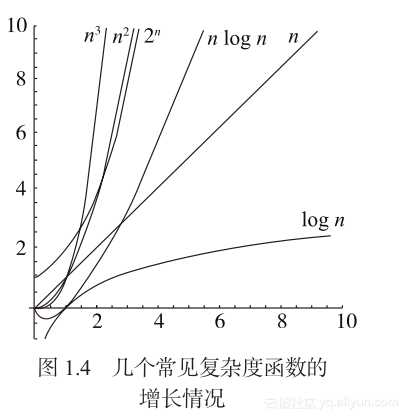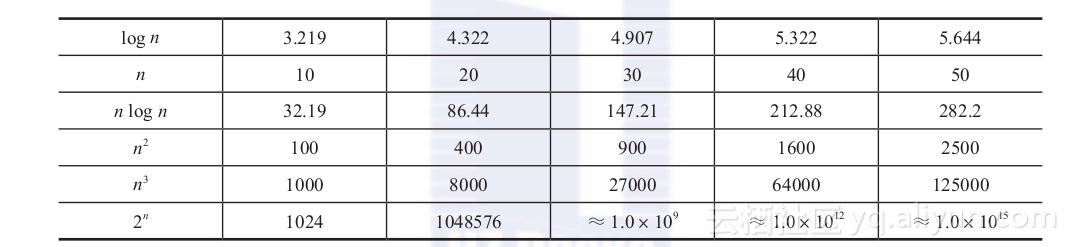# 《数据结构与算法：Python语言描述》一1.3算法和算法分析

+关注继续查看

### 1.3.2算法的代价及其度量

O(1)，O( log n)，O(n)，O(n log n)，O(n2 )，O(n3)，O(2n)def fib(n):
if n < 2:
return 1
else:
return fib(n-1) + fib(n-2)def fib(n):
f1 = f2 = 1
for k in range(1, n):
f1, f2 = f2, f2 + f1
return f2


### 1.3.3算法分析

0.基本操作，认为其时间复杂度为O(1)。如果是函数调用，应该将其时间复杂度代入，参与整体时间复杂度的计算。
1.加法规则（顺序复合）。如果算法（或所考虑算法片段）是两个部分（或多个部分）的顺序复合，其复杂性是这两部分（或多部分）的复杂性之和。以两个部分为例：
T(n)＝T1(n) ＋T2(n)＝O(T1(n))＋O(T2 (n))＝O(max(T1(n), T2(n)))

2.乘法规则（循环结构）。如果算法（或所考虑的算法片段）是一个循环，循环体将执行T1 (n)次，每次执行需要T2 (n)时间，那么：
T (n)＝T1 (n)×T2 (n)＝O (T1 (n))×O (T2 (n))＝O (T1 (n)×T2 (n))
3.取最大规则（分支结构）。如果算法（或所考虑算法片段）是条件分支，两个分支的时间复杂性分别为T1 (n)和T2 (n)，则有：
T (n)＝O (max (T1 (n), T2 (n)))

for i in range(n):
for j in range(n):
x = 0.0
for k in range(n):
x = x + m1[i][k] * m2[k][j]
m[i][j] = x


T(n)＝O(n)×O(n)×(O(1)＋O(n)×O(1)＋O(1))

＝O(n)×O(n)×O(n)＝O(n×n×n)＝O(n3)

# 设被求值矩阵为二维表A0:n

for i in range(n-1):
用Ai将Ai+1:n的值都变为0
det = 0.0
for i in range(n):
det += Ai

T(n)＝n×((n－1)2＋T(n－1))＞n×T(n－1)
＞n×(n－1)×T(n－2)＞O(n!)

def recur(n):
if n == 0:
return g(...)
somework
for i in range(a):
x = recur(n/b)
somework
somework


### 1.3.4Python程序的计算代价（复杂度）

list和tuple的元素访问和元素赋值，是常量时间的。
dict操作的情况比较复杂，后面第8章有详细讨论。

1）Python的各种组合数据对象都没有预设的最大元素个数。在实际使用中，这些结构能根据元素个数的增长自动扩充存储空间。从空间占用的角度看，其实际开销在存续期间可能变大，但通常不会自动缩小（即使后来元素变得很少了）。举个例子，假设程序里建了一个表，而后不断加入元素导致表变得很大，而后又不断删除元素，后来表中元素变得很少，但占用的存储空间并不减少。
2）还应该注意Python自动存储管理系统的影响。举个例子：如果在程序里建立了一个表，此后一直将其作为某个全局变量的值，这个对象就会始终存在并占用存储空间。如果将其作为某个函数里局部变量的值，或者虽然作为全局变量的值，但后来通过赋值将其抛弃，这个表对象就可以被回收。

Python语言提供了很多高级结构，使人很容易通过简短的程序完成很多工作，例如很容易构造出新的表或元组。这种方便性有时也会带来负面作用。懒散而且不警觉的编程者很容易写出一些貌似正确但实际上完全不能用的程序，其时间开销太大而且毫无必要，使程序只能处理很小的实例，基本上不能使用；或者空间开销太大，生成大量不必要的数据对象，直至用完了可用内存，导致程序崩溃。另一个显然的事实是，构造大量数据对象本身也需要时间，这两方面的问题往往是纠缠在一起的。

Python程序的时间复杂度实例

data=[]
while还有数据:

x = 下一数据
data.insert(0,x)#把新数据加在表的最前面


data=[]
while还有数据:
x = 下一数据
data.insert(len(data),x) # 新数据加在最后，或写data.append(x)


def test1(n):
lst=[]
for i in range(n*10000):
lst = lst + [i]
return lst

def test2(n):
lst=[]
for i in range(n*10000):
lst.append(i)
return lst

def test3(n):
return [i for i in range(n*10000)]

def test4(n):
return list(range(n*10000))12 021Python标准库系列之Memcache模块
1071 01Python标准库系列之模块介绍
1155 011Python标准库系列之configparser模块
870 018Python标准库系列之logging模块
1095 022Python标准库系列之Redis模块
1426 07Python标准库系列之requests模块
1318 014Python标准库系列之zipfile模块
1091 0Python标准库系列之模块默认全局变量
945 05Python标准库系列之json模块
1052 0
10057

0

+ 订阅